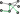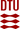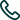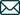# Network Optimization Solver

Leverage mathematical optimization algorithms to solve your network structured mixed integer programmes.

# News

## Ørsted chooses Flowty as their Mathematical Network Optimization Solver

November 12, 2020

Ørsted, the global leader in offshore wind power production and ranked the most sustainable company in the world, has chosen Flowty’s Network Optimization Solver ...

# Why Flowty

Get running fast with intuitive modelling of constrained network problems.

Enjoy specialized algorithms to leverage network structures fully.# What We Do

Solve network optimization problems like

\begin{aligned} \min{ } & \sum_{k \in K} \sum_{e \in E^k} c_e x_e + \sum_{i \in N} f_i y_i \\ \text{s.t. } & \sum_{k \in K} \sum_{e \in E^k} \alpha_{je} x_e + \sum_{i \in N} \beta_{ji} y_i = b & & j \in M \\ & x_e = \sum_{p \in P^k} \Big ( \sum_{ s \in p: s = e} \mathbf{1} \Big ) \lambda_p & & k \in K, e \in E^k \\ & L^k \leq \sum_{p \in P^k} \lambda_p \leq U^k && k \in K \\ & \lambda_p \in \mathbb{Z}^+ && k \in K, p \in P^k \\ & x_e \in \mathbb{Z}, y_i \in \mathbb{Z} && e \in E, i \in N \end{aligned}

for graphs $G(V^k, E^k),\; k \in K$ with paths $p \in P^k$ subject to resource constraints $R^k$.

# How To

Install it

pip install flowty

and solve a time-constrained fixed-charge multi-commodity flow problem

from flowty import Model, xsum

# ... data setup

m = Model()

# create graphs per commodity
g = [
m.addGraph(obj=obj, edges=edges, source=o[k], sink=d[k], L=D[k], U=D[k], type="C")
for k in range(K)
]

# add time constraints to graphs
for k in range(K):

# demand constraints
for k in range(K):
m.addConstr(xsum(x for x in g[k].vars if x.source == g[k].source) == D[k])

# design variables
y = [
for e, edge in enumerate(edges)
]

# capacity constraints
for e, edge in enumerate(edges):
xsum(x for k in range(K) for x in g[k].vars if x.edge == edge) <= u[e] * y[e]
)

status = m.optimize()

Flowty was founded by Simon Spoorendonk, PhD, with the aim to bring easy-to-use network optimization with excellent performance to the masses.

Professor Stefan Røpke, DTU Management, advices Flowty on scientific matters.

# Selected Partners### Contact+4527286731

Flowty ApS, Traps Alle 8, 2500 Valby, Denmark
CVR: 39946408